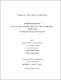## Analysis of a simple gene expression model2012
##### Authors
Chipindirwi, Simbarashe
##### Publisher
Lethbridge, Alta. : University of Lethbridge, Dept. of Chemistry and Biochemistry, c2012
##### Abstract
Gene expression is random owing to the low copy numbers of molecules in a living cell and the best way to study it is by use of a stochastic method, specifically the chemical master equation. The method is used here to derive analytically the invariant probability distributions, and expressions for the moments and noise strength for a simple gene model without feedback. Sensitivity analysis, emphasizing particularly the dependence of the probability distributions, the moments, and noise strength is carried out using Metabolic Control Analysis, which uses control coefficients that measure the response of observables when parameters change. Bifurcation analysis is also carried out. The results show that the number of mRNA molecules follows a hypergeometric probability distribution, and that noise decreases as the number of these molecules increases. Metabolic Control Analysis was successfully extended to genetic control mechanisms, with the obtained control coefficients satisfying a summation theorem. The system undergoes stochastic bifurcations as parameters change.
##### Description
xii, 86 leaves : ill. ; 29 cm
##### Keywords
Gene expression -- Research , Gene expression -- Mathematical models , Stochastic analysis , Markov processes , Dissertations, Academic